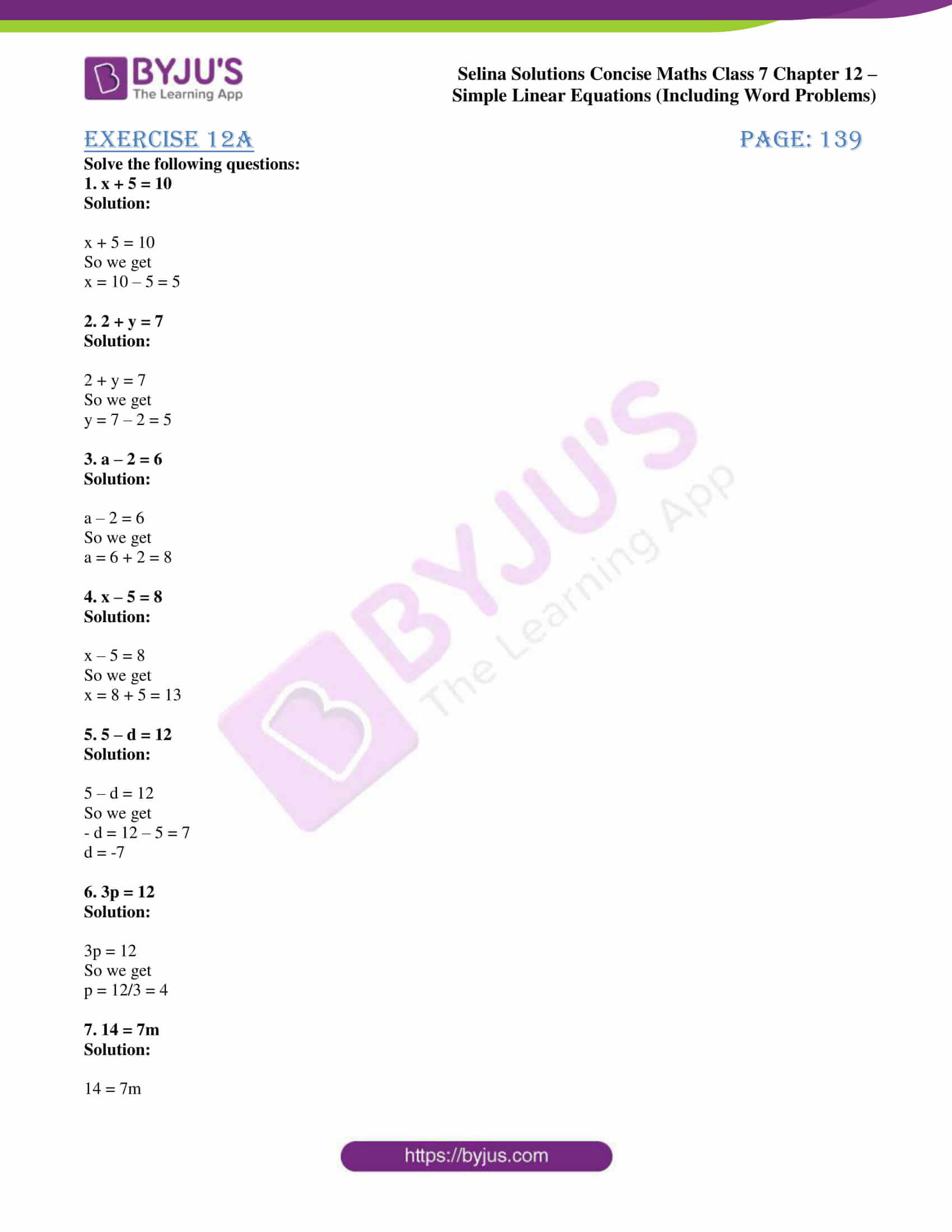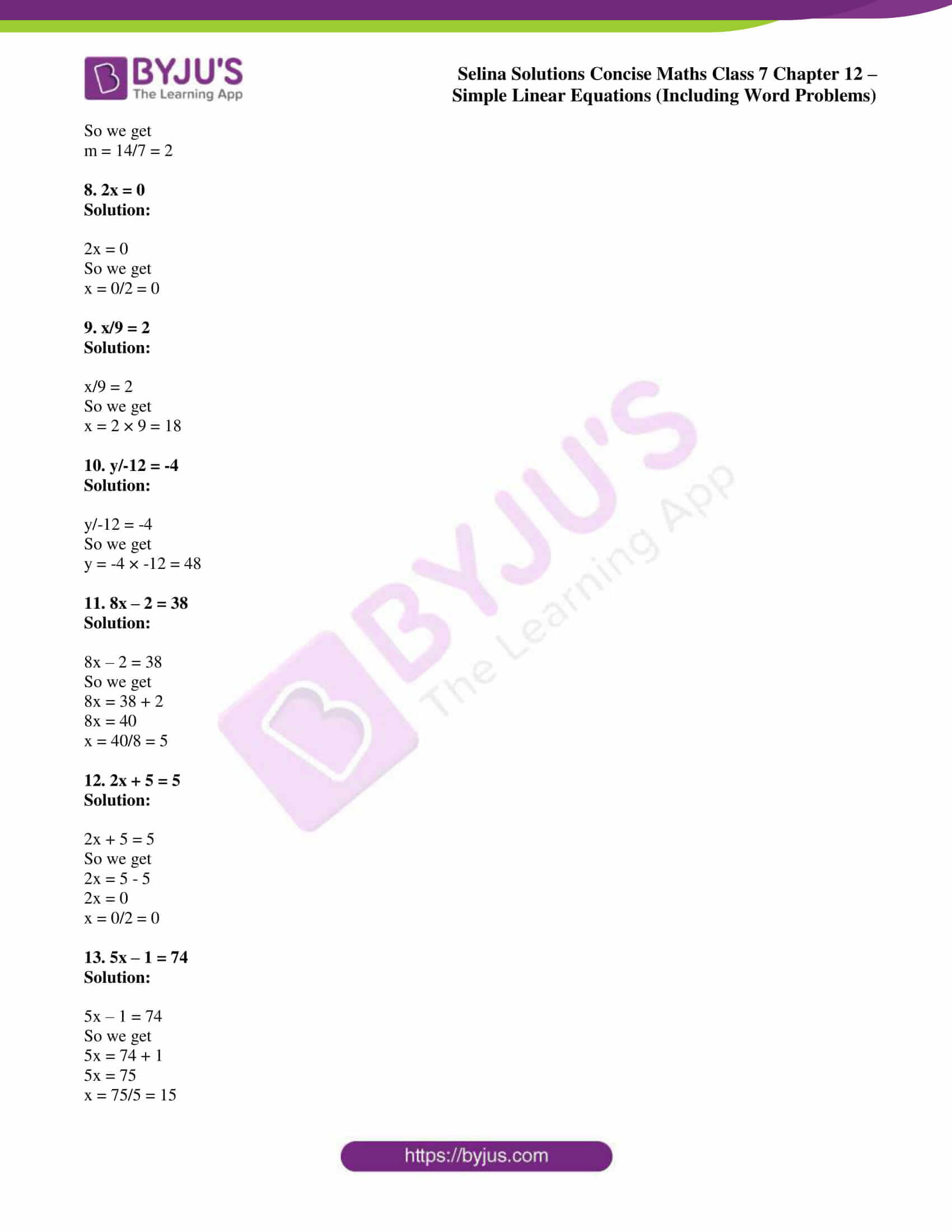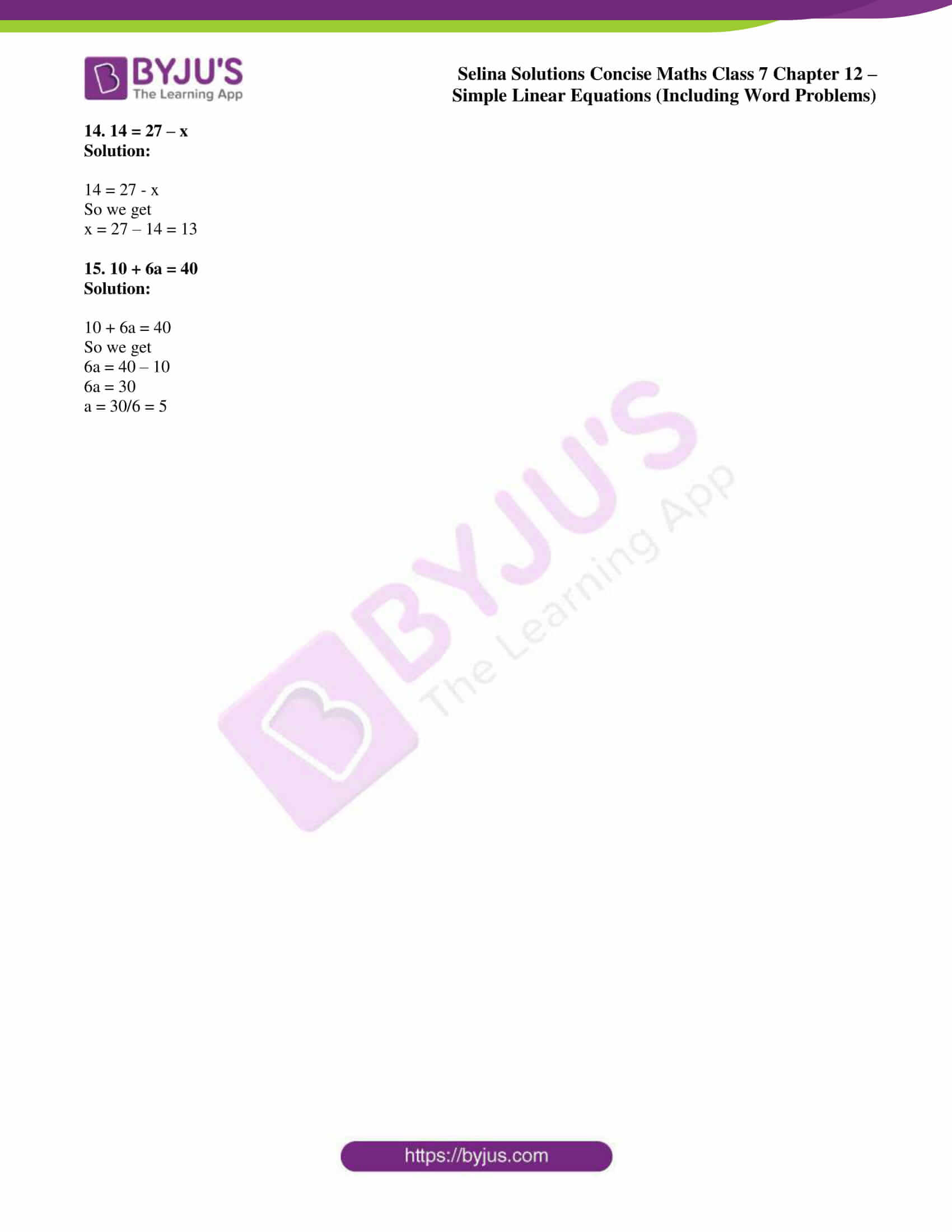# Selina Solutions Concise Maths Class 7 Chapter 12: Simple Linear Equations (Including Word Problems) Exercise 12A

Selina Solutions Concise Maths Class 7 Chapter 12 Simple Linear Equations (Including Word Problems) Exercise 12A contains answers prepared by expert faculty, based on the exam pattern of ICSE board. The solutions are in a stepwise manner as each step carries marks in the annual exam. Our set of faculty at BYJU’S prepare solutions which are 100% accurate to improve the academic performance of students. Selina Solutions Concise Maths Class 7 Chapter 12 Simple Linear Equations (Including Word Problems) Exercise 12A, free PDF links are given below.

## Selina Solutions Concise Maths Class 7 Chapter 12: Simple Linear Equations (Including Word Problems) Exercise 12A Download PDF### Access Selina Solutions Concise Maths Class 7 Chapter 12: Simple Linear Equations (Including Word Problems) Exercise 12A

#### Exercise 12A page: 139

Solve the following questions:

1. x + 5 = 10

Solution:

x + 5 = 10

So we get

x = 10 – 5 = 5

2. 2 + y = 7

Solution:

2 + y = 7

So we get

y = 7 – 2 = 5

3. a – 2 = 6

Solution:

a – 2 = 6

So we get

a = 6 + 2 = 8

4. x – 5 = 8

Solution:

x – 5 = 8

So we get

x = 8 + 5 = 13

5. 5 – d = 12

Solution:

5 – d = 12

So we get

– d = 12 – 5 = 7

d = -7

6. 3p = 12

Solution:

3p = 12

So we get

p = 12/3 = 4

7. 14 = 7m

Solution:

14 = 7m

So we get

m = 14/7 = 2

8. 2x = 0

Solution:

2x = 0

So we get

x = 0/2 = 0

9. x/9 = 2

Solution:

x/9 = 2

So we get

x = 2 × 9 = 18

10. y/-12 = -4

Solution:

y/-12 = -4

So we get

y = -4 × -12 = 48

11. 8x – 2 = 38

Solution:

8x – 2 = 38

So we get

8x = 38 + 2

8x = 40

x = 40/8 = 5

12. 2x + 5 = 5

Solution:

2x + 5 = 5

So we get

2x = 5 – 5

2x = 0

x = 0/2 = 0

13. 5x – 1 = 74

Solution:

5x – 1 = 74

So we get

5x = 74 + 1

5x = 75

x = 75/5 = 15

14. 14 = 27 – x

Solution:

14 = 27 – x

So we get

x = 27 – 14 = 13

15. 10 + 6a = 40

Solution:

10 + 6a = 40

So we get

6a = 40 – 10

6a = 30

a = 30/6 = 5